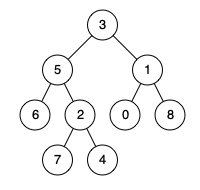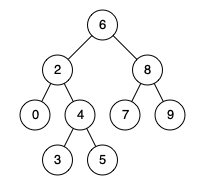# Tree - 109. Convert Sorted List to Binary Search Tree

109. Convert Sorted List to Binary Search Tree Given a singly linked list where elements are sorted in ascending order, convert it to a height balanced BST. For this problem, a height-balanced binary tree is defined as a binary tree in which the depth of the two subtrees of every node never differ by more than 1. Example: Given the sorted linked list: [-10,-3,0,5,9], One possible answer is: [0,-3,9,-10,null,5], which represents the following height balanced BST: 0 / \ -3 9 / / -10....# Tree - 108. Convert Sorted Array to Binary Search Tree Easy

108. Convert Sorted Array to Binary Search Tree Given an array where elements are sorted in ascending order, convert it to a height balanced BST. For this problem, a height-balanced binary tree is defined as a binary tree in which the depth of the two subtrees of every node never differ by more than 1. Example: Given the sorted array: [-10,-3,0,5,9], One possible answer is: [0,-3,9,-10,null,5], which represents the following height balanced BST: 0 / \ -3 9 / / -10 5 思路： 将有序数组变成b....# Tree - 236. Lowest Common Ancestor of a Binary Tree

236. Lowest Common Ancestor of a Binary Tree Given a binary tree, find the lowest common ancestor (LCA) of two given nodes in the tree. According to the definition of LCA on Wikipedia: “The lowest common ancestor is defined between two nodes p and q as the lowest node in T that has both p and q as descendants (where we allow a node to be a descendant of itself).” Given the following binary tree:  root = [3,5,1,6,2,0,8,null,null,7,4]   Example 1: ....# Tree - 235. Lowest Common Ancestor of a Binary Search Tree

235. Lowest Common Ancestor of a Binary Search Tree Given a binary search tree (BST), find the lowest common ancestor (LCA) of two given nodes in the BST. According to the definition of LCA on Wikipedia: “The lowest common ancestor is defined between two nodes p and q as the lowest node in T that has both p and q as descendants (where we allow a node to be a descendant of itself).” Given binary search tree:  root = [6,2,8,0,4,7,9,null,null,3,5]   Example 1....# Tree - 98. Validate Binary Search Tree

98. Validate Binary Search Tree Given a binary tree, determine if it is a valid binary search tree (BST). Assume a BST is defined as follows: The left subtree of a node contains only nodes with keys less than the node’s key. The right subtree of a node contains only nodes with keys greater than the node’s key. Both the left and right subtrees must also be binary search trees.   Example 1: 2 / \ 1 3 Input: [2,1,3] Output: true Example 2: 5 / \ 1 4   / ....# Tree - 199. Binary Tree Right Side View

199. Binary Tree Right Side View Given a binary tree, imagine yourself standing on the right side of it, return the values of the nodes you can see ordered from top to bottom. Example: Input: [1,2,3,null,5,null,4] Output: [1, 3, 4] Explanation: 1 <--- / \ 2 3 <--- \ \ 5 4 <--- 思路： 题目意思是返回树最右边一个不空的节点的值，采用广度优先遍历，遍历每一层，找出最右边的一个节点就可以。 代码： go： /** * Definition for a binary tree node. * type TreeNode struct { * Val int * Left *TreeNode * Right *TreeNode * } */ func....# Tree - 103. Binary Tree Zigzag Level Order Traversal

103. Binary Tree Zigzag Level Order Traversal Given a binary tree, return the zigzag level order traversal of its nodes’ values. (ie, from left to right, then right to left for the next level and alternate between). For example: Given binary tree [3,9,20,null,null,15,7], 3 / \ 9 20 / \ 15 7 return its zigzag level order traversal as: [ , [20,9], [15,7] ] 思路： 简单的层序遍历实现题，思想是bfs，只是在输出结果上用做z字序。 代码： go： /** * Definition for a binary tree node. * type TreeNode struct { * Va....# Tree - 107. Binary Tree Level Order Traversal II

107. Binary Tree Level Order Traversal II Given a binary tree, return the bottom-up level order traversal of its nodes’ values. (ie, from left to right, level by level from leaf to root). For example: Given binary tree [3,9,20,null,null,15,7], 3 / \ 9 20 / \ 15 7 return its bottom-up level order traversal as: [ [15,7], [9,20],  ] 思路： 层序遍历二叉树，倒序输出，很简单的实现题，使用slice当做队列就可以。 代码： go： /** * Definition for a binary tree node. * type TreeNode struct { * Val int * Left *TreeNod....# Trie - 212. Word Search II

212. Word Search II Given a 2D board and a list of words from the dictionary, find all words in the board. Each word must be constructed from letters of sequentially adjacent cell, where “adjacent” cells are those horizontally or vertically neighboring. The same letter cell may not be used more than once in a word.   Example: Input: board = [ ['o','a','a','n'], ['e','t','a','e'], ['i','h','k','r'], ['i','f','l','v'] ] words = ["oath","pea","eat","rain"] Output: ["eat","oath"] Not....# Trie - 211. Add and Search Word - Data structure design# Trie - 208. Implement Trie (Prefix Tree)

208. Implement Trie (Prefix Tree) Implement a trie with insert, search, and startsWith methods. Example: Trie trie = new Trie(); trie.insert("apple"); trie.search("apple"); // returns true trie.search("app"); // returns false trie.startsWith("app"); // returns true trie.insert("app"); trie.search("app"); // returns true Note: You may assume that all inputs are consist of lowercase letters a-z. All inputs are guaranteed to be non-empty strings. 思路： 题目意思很明确，就是实现一下....# Tree - 337. House Robber III

337. House Robber III The thief has found himself a new place for his thievery again. There is only one entrance to this area, called the “root.” Besides the root, each house has one and only one parent house. After a tour, the smart thief realized that “all houses in this place forms a binary tree”. It will automatically contact the police if two directly-linked houses were broken into on the same night. Determine the maximum amount of money the thief can rob tonight without alerting the p....# Tree - 250. Count Univalue Subtrees

250. Count Univalue Subtrees Given a binary tree, count the number of uni-value subtrees. A Uni-value subtree means all nodes of the subtree have the same value. Example Example1 Input: root = {5,1,5,5,5,#,5} Output: 4 Explanation: 5 / \ 1 5 / \ \ 5 5 5 Example2 Input: root = {1,3,2,4,5,#,6} Output: 3 Explanation: 1 / \ 3 2 / \ \ 4 5 6 思路： 题目意思是找出一棵树中以树中某节点为根节点的子树中所有元素都相同，很明显，叶子结点肯定是一个uni的子树，回到问题本身，判断以一个节点为根节点的子树是否为uni-value，只需要满足左子树是uni-value，右子树是uni-value，并且判断当前节点和左右孩子节点值，就能求解。所以，递归后序遍....# Tree - 124. Binary Tree Maximum Path Sum

124. Binary Tree Maximum Path Sum Given a non-empty binary tree, find the maximum path sum. For this problem, a path is defined as any sequence of nodes from some starting node to any node in the tree along the parent-child connections. The path must contain at least one node and does not need to go through the root. Example 1: Input: [1,2,3] 1 / \ 2 3 Output: 6 Example 2: Input: [-10,9,20,null,null,15,7]   -10    / \   9  20     / &nb....# Tree - 110. Balanced Binary Tree

110. Balanced Binary Tree Given a binary tree, determine if it is height-balanced. For this problem, a height-balanced binary tree is defined as: a binary tree in which the depth of the two subtrees of every node never differ by more than 1. Example 1: Given the following tree [3,9,20,null,null,15,7]: 3 / \ 9 20 / \ 15 7 Return true. Example 2: Given the following tree [1,2,2,3,3,null,null,4,4]: 1 / \ 2 2 / \ 3 3 / \ 4 4 Return false. 思路： 题目意思是判断一棵二叉树是否是平衡二叉树。而平衡二....# Tree - 104. Maximum Depth of Binary Tree

104. Maximum Depth of Binary Tree Given a binary tree, find its maximum depth. The maximum depth is the number of nodes along the longest path from the root node down to the farthest leaf node. Note: A leaf is a node with no children. Example: Given binary tree [3,9,20,null,null,15,7], 3 / \ 9 20 / \ 15 7 return its depth = 3. 思路： 一棵空树的深度为零，也就是可以看做叶子节点的孩子节点的深度为零，叶子节点的深度为1，而一个树的深度和高度相同，所以求一个树的深度其实就是左右子树的深度的最大值加一。递归求解就可以。 代码： go： /** * Definition for a binary tree node. * type T....# Tree - 298. Binary Tree Longest Consecutive Sequence

298 . Binary Tree Longest Consecutive Sequence Description Given a binary tree, find the length of the longest consecutive sequence path. The path refers to any sequence of nodes from some starting node to any node in the tree along the parent-child connections. The longest consecutive path need to be from parent to child (cannot be the reverse). Example 1: Input: 1 \ 3 / \ 2 4 \ 5 Output:3 Explanation: Longest consecutive sequence path is 3-4-5, so return 3. 思路： 找出根到叶子节点的路径中的最长递增序列，做法就是由根节点向叶....# Tree - 111. Minimum Depth of Binary Tree

111. Minimum Depth of Binary Tree Given a binary tree, find its minimum depth. The minimum depth is the number of nodes along the shortest path from the root node down to the nearest leaf node. Note: A leaf is a node with no children. Example: Given binary tree [3,9,20,null,null,15,7], 3 / \ 9 20 / \ 15 7 return its minimum depth = 2. 思路： 求二叉树的最小深度，可以采用深度优先搜索递归求解。 代码： go： /** * Definition for a binary tree node. * type TreeNode struct { * Val int * Left *TreeNode * Right ....# Tree - 129. Sum Root to Leaf Numbers

129. Sum Root to Leaf Numbers Given a binary tree containing digits from 0-9 only, each root-to-leaf path could represent a number. An example is the root-to-leaf path 1->2->3 which represents the number 123. Find the total sum of all root-to-leaf numbers. Note: A leaf is a node with no children. Example: Input: [1,2,3] 1 / \ 2 3 Output: 25 Explanation: The root-to-leaf path 1->2 represents the number 12. The root-to-leaf path 1->3 represents the nu....# Tree - 113. Path Sum II

113. Path Sum II Given a binary tree and a sum, find all root-to-leaf paths where each path’s sum equals the given sum. Note: A leaf is a node with no children. Example: Given the below binary tree and sum = 22, 5 / \ 4 8 / / \ 11 13 4 / \ / \ 7 2 5 1 Return: [ [5,4,11,2], [5,8,4,5] ] 思路： 题目意思是求出所有根节点到叶子结点路径和为sum的结果。递归求解，找到叶子节点比较sum就可以 代码： go： /** * Definition for a binary tree node. * type TreeNode struct { * Val int * Left *TreeNode * Right *TreeNode * } */ func pathSum(roo....

Nothing just happens, it's all part of a plan.